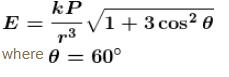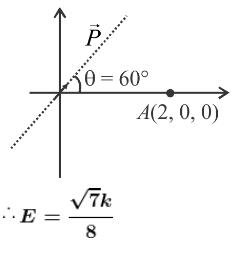Courses

# Electrostatics NAT Level - 1

## 10 Questions MCQ Test Topic wise Tests for IIT JAM Physics | Electrostatics NAT Level - 1

Description
This mock test of Electrostatics NAT Level - 1 for Physics helps you for every Physics entrance exam. This contains 10 Multiple Choice Questions for Physics Electrostatics NAT Level - 1 (mcq) to study with solutions a complete question bank. The solved questions answers in this Electrostatics NAT Level - 1 quiz give you a good mix of easy questions and tough questions. Physics students definitely take this Electrostatics NAT Level - 1 exercise for a better result in the exam. You can find other Electrostatics NAT Level - 1 extra questions, long questions & short questions for Physics on EduRev as well by searching above.
*Answer can only contain numeric values
QUESTION: 1

### A mercury drop of water has potential V on its surface. 1000 such drops combine to form a new drop. Find the potential (in Volts) on the surface of the new drop.

Solution:

Let the radius of each mercury drop be r.
If q is charge on each drop
The potential of drop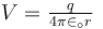or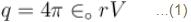Let R be the radius of the new drop formed by combination of 1000 drops of radius r.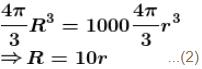∴ Potential of new drop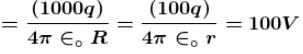*Answer can only contain numeric values
QUESTION: 2

### A charged particle q is placed at the centre O of cube (ABCDEFGH) of length  L. Another same charge q is placed at a distance L from O. Then the electric flux through (BCFG)  is :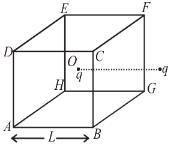Solution:

Total flux in = Total flux out
∴ Net flux through BCFG = 0

*Answer can only contain numeric values
QUESTION: 3

### The number of electrons to be put on spherical conductor of radius 0.1m, to produce an electric field of 0.036N/C just above the surface is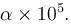Find the value of  α

Solution:

The electric field at the surface of conductor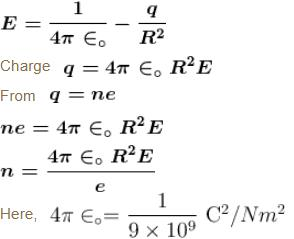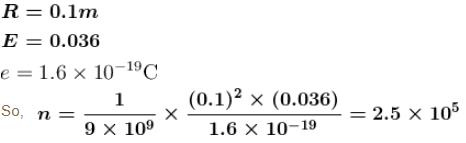*Answer can only contain numeric values
QUESTION: 4

At distance of 5cm and 10cm outwards from the surface of a uniformly charged solid sphere, the potentials are 100V and 75V respectively. Then charge on the sphere is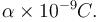Find the Value of  α.

Solution: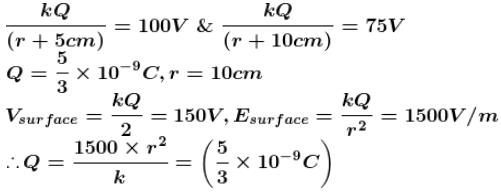*Answer can only contain numeric values
QUESTION: 5

Two positive point charges each of magnitude 10C are fixed at positions A and B at a separation 2d = 6m.  A negatively charged particle of mass m = 90gm and charge of magnitude 10 × 105C  is revolving in a circular path of radius 4m in the plane perpendicular to the line AB and bisecting the line AB. Neglect the effect of gravity. If the angular velocity of the particle is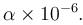Then the value of α (in rad/sec) is :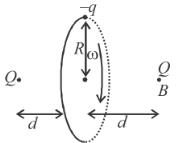Solution:

Net force on –q towards the centre,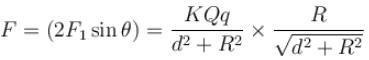For particle to move in circle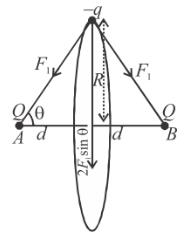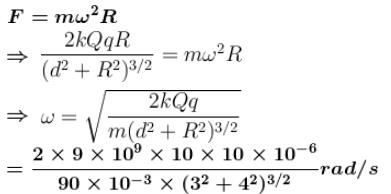*Answer can only contain numeric values
QUESTION: 6

Eight point charges (can be assumed as small sphere uniformly charged and their centres at the corner of the cube) having values q each are fixed at vertices of a cube. The electric flux through square surface ABCD of the cube is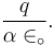Find the value of α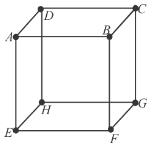Solution:

The electric flux through ABCD due to charges at ABC and D is zero.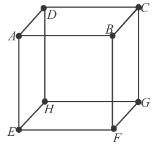The flux through ABCD due to any of the charges at EFG and H are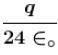each.

Hence the net electric flux through ABCD is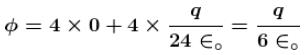*Answer can only contain numeric values
QUESTION: 7

Figure given, shows a closed Gaussian surface in the shape of a cube of edge length 3.0m. There exists an electric field given by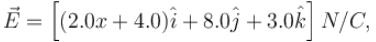where x is in metres, in the region in which it lies. The net charge in coulombs enclosed by the cube is equal to α∈0. Find the value of α.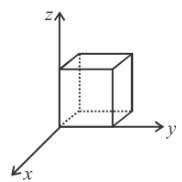Solution: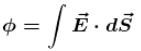Direction of field at x = –3m is along negative x-axis. Area vector is also along same direction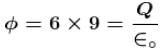Components of electric field which are constant, do not contribute in net flux in or out.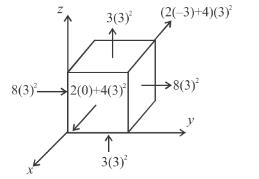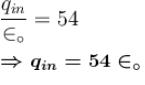*Answer can only contain numeric values
QUESTION: 8

A square loop of side ℓ having uniform linear charge density λ is placed in xy plane as shown in the figure. There is a non-uniform electric field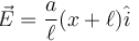where a  is a constant. Then resultant electric force on the loop if ℓ = 10 cm, a = 2N/C and charge density λ = 2μC/m is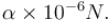Find the value of α.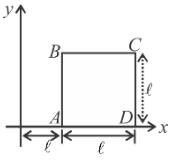Solution: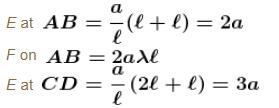on BC  and  AD electric field is no uniform
∴  x is not constant. But on BC and AD electric field will have the same type of variation.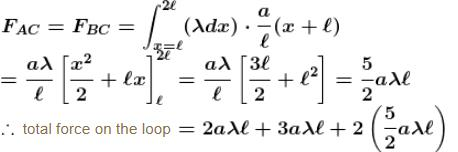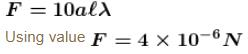*Answer can only contain numeric values
QUESTION: 9

On moving a charge of 20coulombs by 2cm, 2J of work is done, then the potential difference (in Volts) between the points is :

Solution:

By definition of electrostatic potential energy,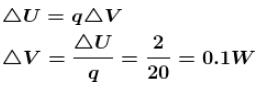*Answer can only contain numeric values
QUESTION: 10

The magnitude of electric field intensity at point (2, 0, 0) due to a dipole moment,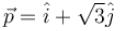kept at origin is αk [where k = 1/π ∈0]. Find the value of α.

Solution:

The dipole moment makes an angle 60° with x-axis and lies in x-y plane as shown in figure.
The electric field a point A due to dipole is